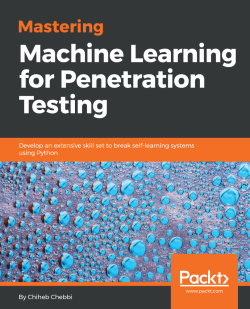# Mastering Machine Learning for Penetration Testing

By Chiheb Chebbi
• \$33.99 eBook
• \$41.99 Print + eBook
What do you get with a Packt Subscription?

• Instant access to this title and 7,500+ eBooks & Videos
• Constantly updated with 100+ new titles each month
• Breadth and depth in over 1,000+ technologies

Cyber security is crucial for both businesses and individuals. As systems are getting smarter, we now see machine learning interrupting computer security. With the adoption of machine learning in upcoming security products, it’s important for pentesters and security researchers to understand how these systems work, and to breach them for testing purposes.

This book begins with the basics of machine learning and the algorithms used to build robust systems. Once you’ve gained a fair understanding of how security products leverage machine learning, you'll dive into the core concepts of breaching such systems. Through practical use cases, you’ll see how to find loopholes and surpass a self-learning security system.

As you make your way through the chapters, you’ll focus on topics such as network intrusion detection and AV and IDS evasion. We’ll also cover the best practices when identifying ambiguities, and extensive techniques to breach an intelligent system.

By the end of this book, you will be well-versed with identifying loopholes in a self-learning security system and will be able to efficiently breach a machine learning system.

Publication date:
June 2018
Publisher
Packt
Pages
276
ISBN
9781788997409

# Introduction to Machine Learning in Pentesting

Currently, machine learning techniques are some of the hottest trends in information technology. They impact every aspect of our lives, and they affect every industry and field. Machine learning is a cyber weapon for information security professionals. In this book, readers will not only explore the fundamentals behind machine learning techniques, but will also learn the secrets to building a fully functional machine learning security system. We will not stop at building defensive layers; we will illustrate how to build offensive tools to attack and bypass security defenses. By the end of this book, you will be able to bypass machine learning security systems and use the models constructed in penetration testing (pentesting) missions.

In this chapter, we will cover:

• Machine learning models and algorithms
• Performance evaluation metrics
• Dimensionality reduction
• Ensemble learning
• Machine learning development environments and Python libraries
• Machine learning in penetration testing – promises and challenges

# Artificial intelligence and machine learning

Making a machine think like a human is one of the oldest dreams. Machine learning techniques are used to help make predictions based on experiences and data.

# Machine learning models and algorithms

In order to teach machines how to solve a large number of problems by themselves, we need to consider the different machine learning models. As you know, we need to feed the model with data; that is why machine learning models are divided, based on datasets entered (input), into four major categories: supervised learning, semi-supervised learning, unsupervised learning, and reinforcement. In this section, we are going to describe each model in a detailed way, in addition to exploring the most well-known algorithms used in every machine learning model. Before building machine learning systems, we need to know how things work underneath the surface.

# Supervised

We talk about supervised machine learning when we have both the input variables and the output variables. In this case, we need to map the function (or pattern) between the two parties. The following are some of the most often used supervised machine learning algorithms.

# Bayesian classifiers

According to the Cambridge English Dictionary, bias is the action of supporting or opposing a particular person or thing in an unfair way, allowing personal opinions to influence your judgment. Bayesian machine learning refers to having a prior belief, and updating it later by using data. Mathematically, it is based on the Bayes formula:One of the simplest Bayesian problems is randomly tossing a coin and trying to predict whether the output will be heads or tails. That is why we can identify Bayesian methodology as being probabilistic. Naive Bayes is very useful when you are using a small amount of data.

# Support vector machines

A support vector machine (SVM) is a supervised machine learning model that works by identifying a hyperplane between represented data. The data can be represented in a multidimensional space. Thus, SVMs are widely used in classification models. In an SVM, the hyperplane that best separates the different classes will be used. In some cases, when we have different hyperplanes that separate different classes, identification of the correct one will be performed thanks to something called a margin, or a gap. The margin is the nearest distance between the hyperplanes and the data positions. You can take a look at the following representation to check for the margin: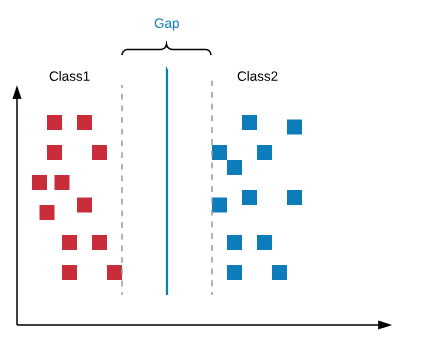The hyperplane with the highest gap will be selected. If we choose the hyperplane with the shortest margin, we might face misclassification problems later. Don't be distracted by the previous graph; the hyperplane will not always be linear. Consider a case like the following: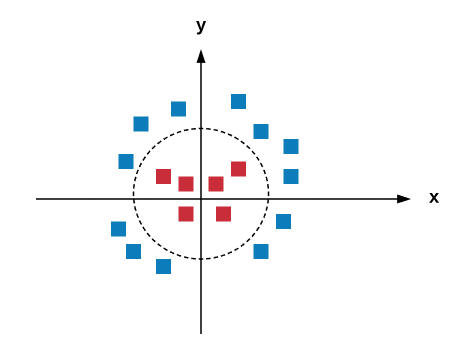In the preceding situation, we can add a new axis, called the z axis, and apply a transformation using a kernel trick called a kernel function, where z=x^2+y^2. If you apply the transformation, the new graph will be as follows: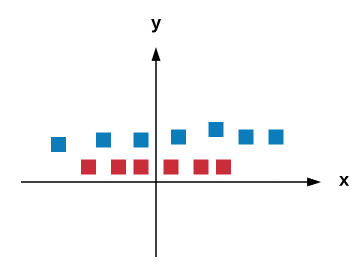Now, we can identify the right hyperplane. The transformation is called a kernel. In the real world, finding a hyperplane is very hard. Thus, two important parameters, called regularization and gamma, play a huge role in the determination of the right hyperplane, and in every SVM classifier to obtain better accuracy in nonlinear hyperplane situations.

# Decision trees

Decision trees are supervised learning algorithms used in decision making by representing data as trees upside-down with their roots at the top. The following is a graphical representation of a decision tree: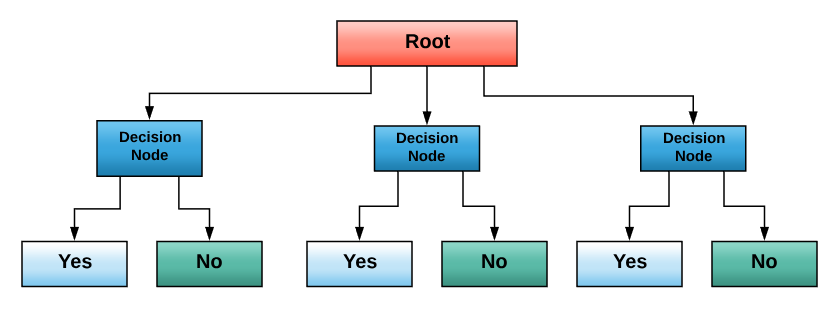Data is represented thanks to the Iterative Dichotomiser 3 algorithm. Decision trees used in classification and regression problems are called CARTs. They were introduced by Leo Breiman.

# Semi-supervised

Semi-supervised learning is an area between the two previously discussed models. In other words, if you are in a situation where you are using a small amount of labeled data in addition to unlabeled data, then you are performing semi-supervised learning. Semi-supervised learning is widely used in real-world applications, such as speech analysis, protein sequence classification, and web content classification. There are many semi-supervised methods, including generative models, low-density separation, and graph-based methods (discrete Markov Random Fields, manifold regularization, and mincut).

# Unsupervised

In unsupervised learning, we don't have clear information about the output of the models. The following are some well-known unsupervised machine learning algorithms.

# Artificial neural networks

Artificial networks are some of the hottest applications in artificial intelligence, especially machine learning. The main aim of artificial neural networks is building models that can learn like a human mind; in other words, we try to mimic the human mind. That is why, in order to learn how to build neural network systems, we need to have a clear understanding of how a human mind actually works. The human mind is an amazing entity. The mind is composed and wired by neurons. Neurons are responsible for transferring and processing information.

We all know that the human mind can perform a lot of tasks, like hearing, seeing, tasting, and many other complicated tasks. So logically, one might think that the mind is composed of many different areas, with each area responsible for a specific task, thanks to a specific algorithm. But this is totally wrong. According to research, all of the different parts of the human mind function thanks to one algorithm, not different algorithms. This hypothesis is called the one algorithm hypothesis.

Now we know that the mind works by using one algorithm. But what is this algorithm? How is it used? How is information processed with it?

To answer the preceding questions, we need to look at the logical representation of a neuron. The artificial representation of a human neuron is called a perceptron. A perceptron is represented by the following graph: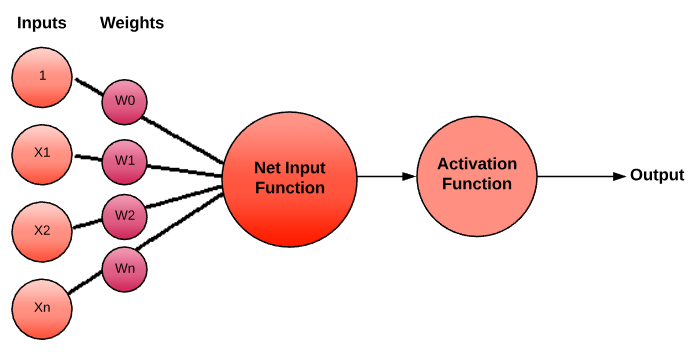There are many Activation Functions used. You can view them as logical gates:

• Step function: A predefined threshold value.
• Sigmoid function: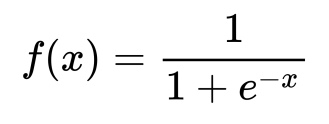• Tanh function: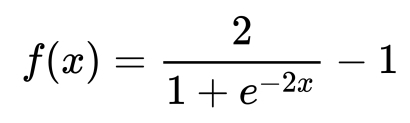• ReLu function:Many fully connected perceptrons comprise what we call a Multi-Layer Perceptron (MLP) network. A typical neural network contains the following:

• An input layer
• Hidden layers
• Output layers

We will discuss the term deep learning once we have more than three hidden layers. There are many types of deep learning networks used in the world:

• Convolutional neural networks (CNNs)
• Recursive neural networks (RNNs)
• Long short-term memory (LSTM)
• Shallow neural networks
• Autoencoders (AEs)
• Restricted Boltzmann machines

Don't worry; we will discuss the preceding algorithms in detail in future chapters.

To build deep learning models, we follow five steps, suggested by Dr. Jason Brownlee. The five steps are as follows:

1. Network definition
2. Network compiling
3. Network fitting
4. Network evaluation
5. Prediction

# Linear regression

Linear regression is a statistical and machine learning technique. It is widely used to understand the relationship between inputs and outputs. We use linear regression when we have numerical values.

# Logistic regression

Logistic regression is also a statistical and machine learning technique, used as a binary classifier - in other words, when the outputs are classes (yes/no, true/false, 0/1, and so on).

# Clustering with k-means

k-Nearest Neighbors (kNN) is a well-known clustering method. It is based on finding similarities in data points, or what we call the feature similarity. Thus, this algorithm is simple, and is widely used to solve many classification problems, like recommendation systems, anomaly detection, credit ratings, and so on . However, it requires a high amount of memory. While it is a supervised learning model, it should be fed by labeled data, and the outputs are known. We only need to map the function that relates the two parties. A kNN algorithm is non-parametric. Data is represented as feature vectors. You can see it as a mathematical representation: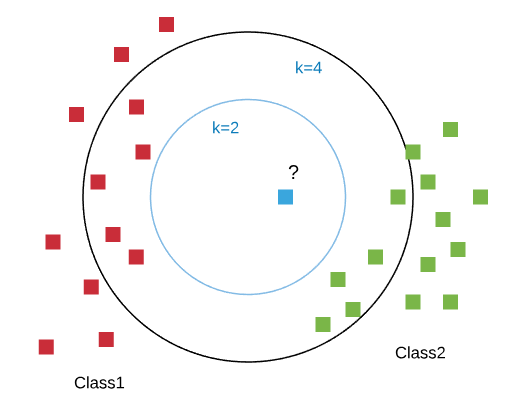The classification is done like a vote; to know the class of the data selected, you must first compute the distance between the selected item and the other, training item. But how can we calculate these distances?

Generally, we have two major methods for calculating. We can use the Euclidean distance: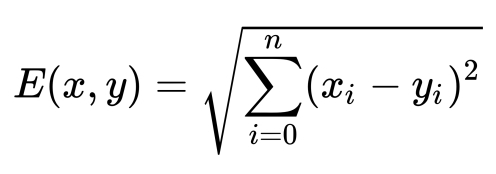Or, we can use the cosine similarity: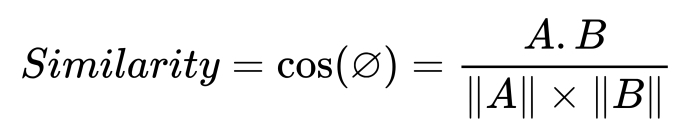The second step is choosing k the nearest distances (k can be picked arbitrarily). Finally, we conduct a vote, based on a confidence level. In other words, the data will be assigned to the class with the largest probability.

# Reinforcement

In the reinforcement machine learning model, the agent is in interaction with its environment, so it learns from experience, by collecting data during the process; the goal is optimizing what we call a long term reward. You can view it as a game with a scoring system. The following graph illustrates a reinforcement model: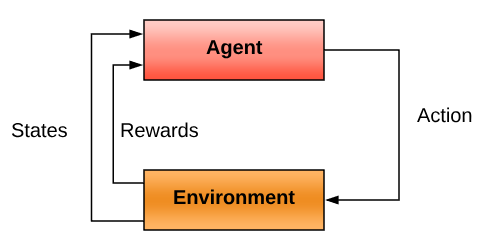# Performance evaluation

Evaluation is a key step in every methodological operation. After building a product or a system, especially a machine learning model, we need to have a clear vision about its performance, to make sure that it will act as intended later on. In order to evaluate a machine learning performance, we need to use well-defined parameters and insights. To compute the different evaluation metrics, we need to use four important parameters:

• True positive
• False positive
• True negative
• False negative

The notations for the preceding parameters are as follows:

• tp: True positive
• fp: False positive
• tn: True negative
• fn: False negative

There are many machine learning evaluation metrics, such as the following:

• Precision: Precision, or positive predictive value, is the ratio of positive samples that are correctly classified divided by the total number of positive classified samples: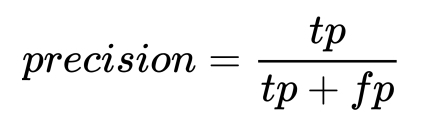• Recall: Recall, or the true positive rate, is the ratio of true positive classifications divided by the total number of positive samples in the dataset: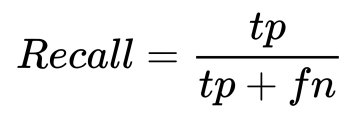• F-Score: The F-score, or F-measure, is a measure that combines the precision and recall in one harmonic formula: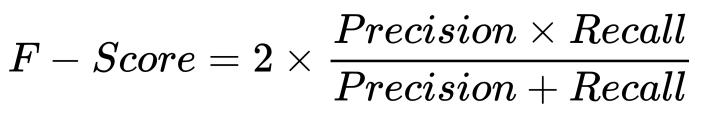• Accuracy: Accuracy is the ratio of the total correctly classified samples divided by the total number of samples. This measure is not sufficient by itself, because it is used when we have an equal number of classes.
• Confusion matrix: The confusion matrix is a graphical representation of the performance of a given machine learning model. It summarizes the performance of each class in a classification problem.

# Dimensionality reduction

Dimensionality reduction is used to reduce the dimensionality of a dataset. It is really helpful in cases where the problem becomes intractable, when the number of variables increases. By using the term dimensionality, we are referring to the features. One of the basic reduction techniques is feature engineering.

Generally, we have many dimensionality reduction algorithms:

• Low variance filter: Dropping variables that have low variance, compared to others.
• High correlation filter: This identifies the variables with high correlation, by using pearson or polychoric, and selects one of them using the Variance Inflation Factor (VIF).
• Backward feature elimination: This is done by computing the sum of square of error (SSE) after eliminating each variable n times.
• Linear Discriminant Analysis (LDA): This reduces the number of dimensions, n, from the original to the number of classes — 1 number of features.
• Principal Component Analysis (PCA): This is a statistical procedure that transforms variables into a new set of variables (principle components).

# Improving classification with ensemble learning

In many cases, when you build a machine learning model, you receive low accuracy and low results. In order to get good results, we can use ensemble learning techniques. This can be done by combining many machine learning techniques into one predictive model.

We can categorize ensemble learning techniques into two categories:

• Parallel ensemble methods—The following graph illustrates how parallel ensemble learning works: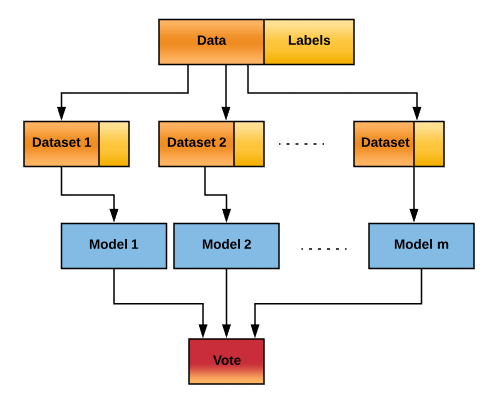• Sequential ensemble methods—The following graph illustrates how sequential ensemble learning works: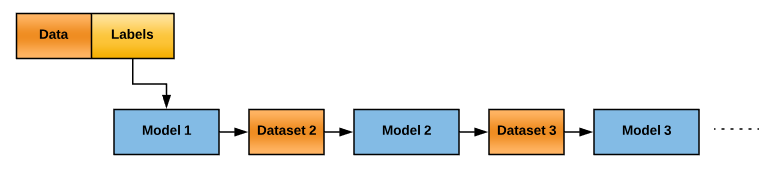The following are the three most used ensemble learning techniques:

• Bootstrap aggregating (bagging): This involves building separate models and combining them by using model averaging techniques, like weighted average and majority vote.
• Boosting: This is a sequential ensemble learning technique. Gradient boosting is one of the most used boosting techniques.
• Stacking: This is like boosting, but it uses a new model to combine submodels.

# Machine learning development environments and Python libraries

At this point, we have acquired knowledge about the fundamentals behind the most used machine learning algorithms. Starting with this section, we will go deeper, walking through a hands-on learning experience to build machine learning-based security projects. We are not going to stop there; throughout the next chapters, we will learn how malicious attackers can bypass intelligent security systems. Now, let's put what we have learned so far into practice. If you are reading this book, you probably have some experience with Python. Good for you, because you have a foundation for learning how to build machine learning security systems.

I bet you are wondering, why Python? This is a great question. According to the latest research, Python is one of the most, if not the most, used programming languages in data science, especially machine learning. The most well-known machine learning libraries are for Python. Let's discover the Python libraries and utilities required to build a machine learning model.

# NumPy

The numerical Python library is one of the most used libraries in mathematics and logical operations on arrays. It is loaded with many linear algebra functionalities, which are very useful in machine learning. And, of course, it is open source, and is supported by many operating systems.

To install NumPy, use the pip utility by typing the following command:

`#pip install numpy`

Now, you can start using it by importing it. The following script is a simple array printing example: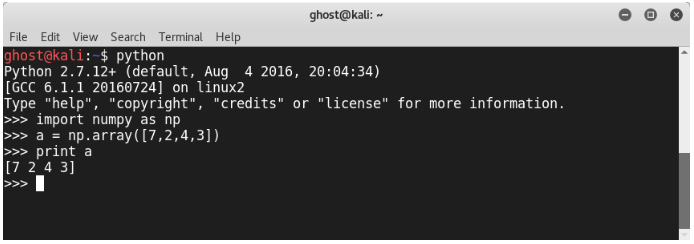In addition, you can use a lot of mathematical functions, like cosine, sine, and so on.

# SciPy

Scientific Python (SciPy) is like NumPy—an amazing Python package, loaded with a large number of scientific functions and utilities. For more details, you can visit https://www.scipy.org/getting-started.html: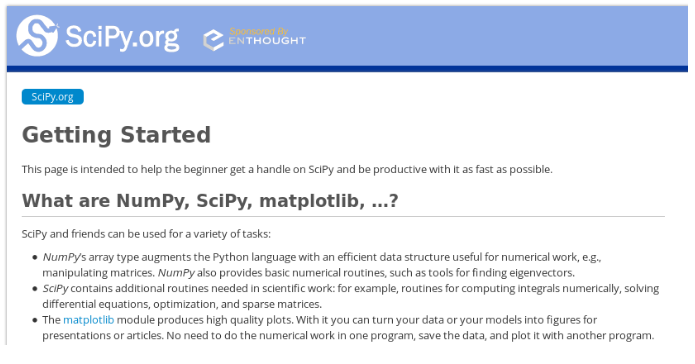# TensorFlow

If you have been into machine learning for a while, you will have heard of TensorFlow, or have even used it to build a machine learning model or to feed artificial neural networks. It is an amazing open source project, developed essentially and supported by Google:The following is the main architecture of TensorFlow, according to the official website: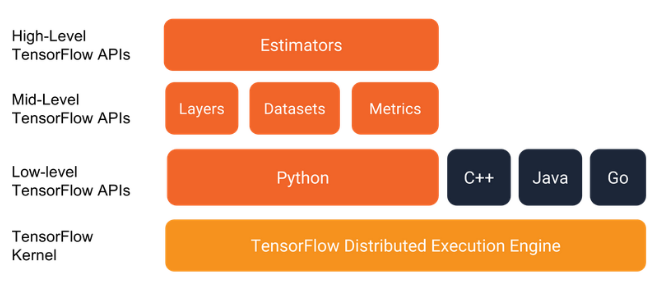If it is your first time using TensorFlow, it is highly recommended to visit the project's official website at https://www.tensorflow.org/get_started/. Let's install it on our machine, and discover some of its functionalities. There are many possibilities for installing it; you can use native PIP, Docker, Anaconda, or Virtualenv.

Let's suppose that we are going to install it on an Ubuntu machine (it also supports the other operating systems). First, check your Python version with the python --version command: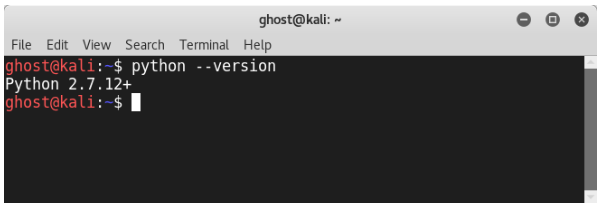Install PIP and Virtualenv using the following command:

`sudo apt-get install python-pip python-dev python-virtualenv`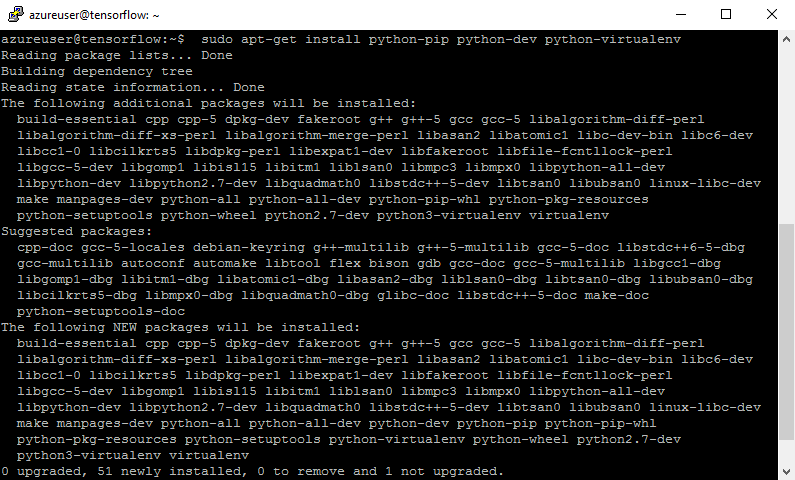Now, the packages are installed: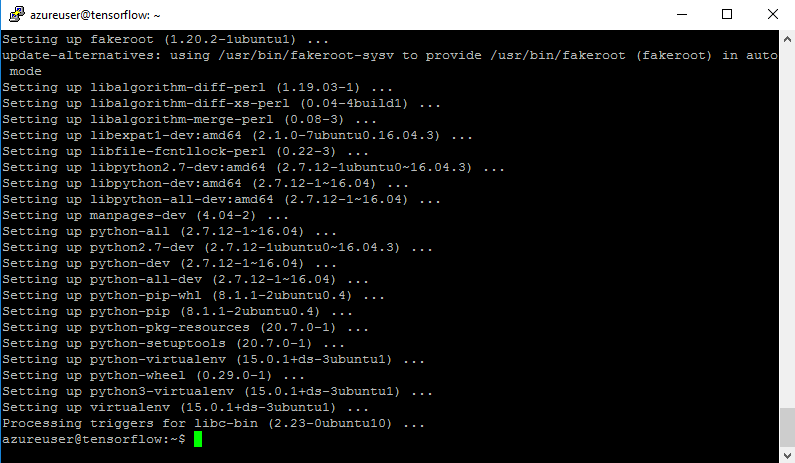Create a new repository using the mkdir command:

`#mkdir TF-project`

Create a new Virtualenv by typing the following command:

` virtualenv --system-site-packages TF-project`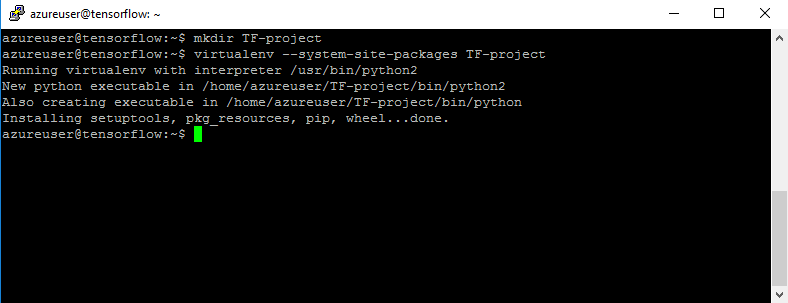Then, type the following command:

`source  <Directory_Here>/bin/activate`

Upgrade TensorFlow by using the pip install -upgrade tensorflow command: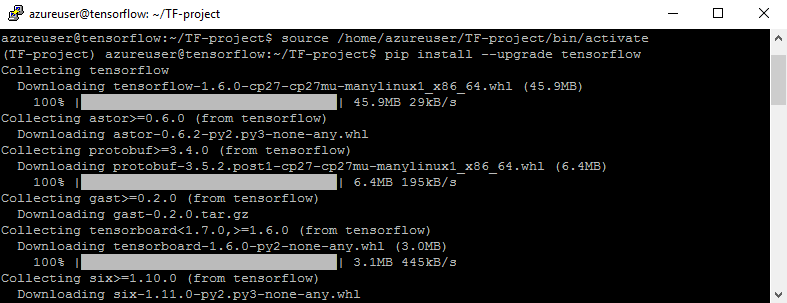`>>> import tensorflow as tf>>> Message = tf.constant("Hello, world!")>>> sess = tf.Session()>>> print(sess.run(Message))`

The following are the full steps to display a Hello World! message: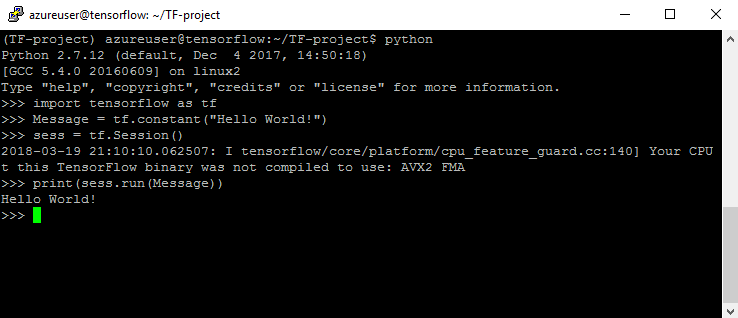# Keras

Keras is a widely used Python library for building deep learning models. It is so easy, because it is built on top of TensorFlow. The best way to build deep learning models is to follow the previously discussed steps:

2. Defining the model
3. Compiling the model
4. Fitting
5. Evaluation
6. Prediction

Before building the models, please ensure that SciPy and NumPy are preconfigured. To check, open the Python command-line interface and type, for example, the following command, to check the NumPy version:

` >>>print numpy.__version__`

To install Keras, just use the PIP utility:

`\$ pip install keras`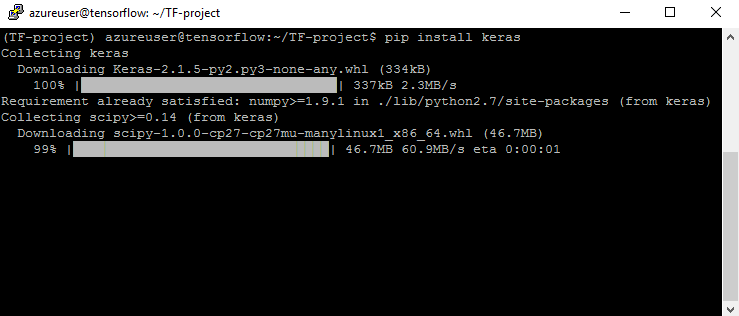And of course to check the version, type the following command:

`>>> print keras.__version__`

To import from Keras, use the following:

`from keras import [what_to_use]from keras.models import Sequentialfrom keras.layers import Dense`

Now, we need to load data:

`dataset = numpy.loadtxt("DATASET_HERE", delimiter=",")I = dataset[:,0:8]O = dataset[:,8]  #the data is splitted into Inputs (I) and Outputs (O)`

You can use any publicly available dataset. Next, we need to create the model:

`model = Sequential()# N = number of neurons# V = number of variablemodel.add(Dense(N, input_dim=V, activation='relu'))# S = number of neurons in the 2nd layermodel.add(Dense(S, activation='relu'))model.add(Dense(1, activation='sigmoid')) # 1 output`

Now, we need to compile the model:

`model.compile(loss='binary_crossentropy', optimizer='adam', metrics=['accuracy'])`

And we need to fit the model:

`model.fit(I, O, epochs=E, batch_size=B)`

As discussed previously, evaluation is a key step in machine learning; so, to evaluate our model, we use:

`scores = model.evaluate(I, O)print("\n%s: %.2f%%" % (model.metrics_names, scores*100))`

To make a prediction, add the following line:

`predictions = model.predict(Some_Input_Here)`

# pandas

pandas is an open source Python library, known for its high performance; it was developed by Wes McKinney. It quickly manipulates data. That is why it is widely used in many fields in academia and commercial activities. Like the previous packages, it is supported by many operating systems.

To install it on an Ubuntu machine, type the following command:

`sudo apt-get install python-pandas`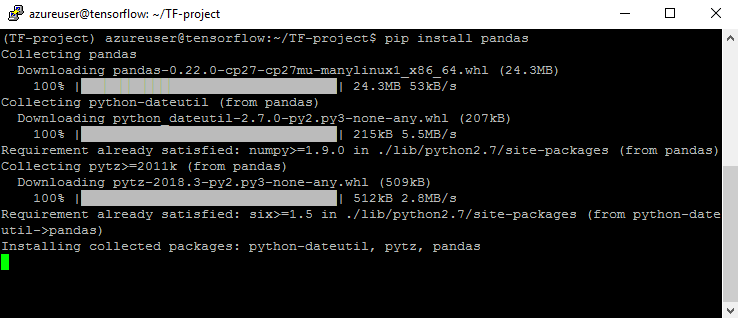Basically, it manipulates three major data structures - data frames, series, and panels:

`>> import pandas as pd>>>import numpy as np data = np.array(['p','a','c','k',’t’]) SR = pd.Series(data) print SR`

I resumed all of the previous lines in this screenshot: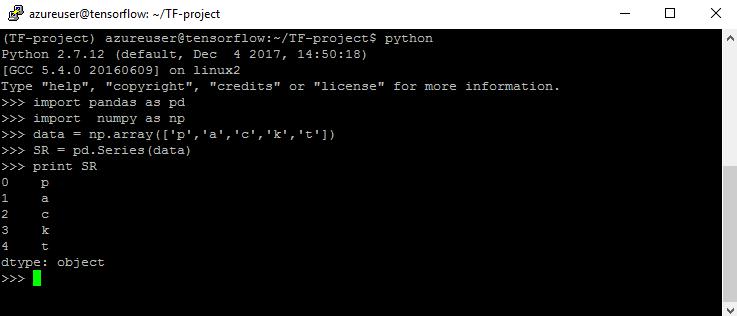# Matplotlib

As you know, visualization plays a huge role in gaining insights from data, and is also very important in machine learning. Matplotlib is a visualization library used for plotting by data scientists. You can get a clearer understanding by visiting its official website at https://matplotlib.org: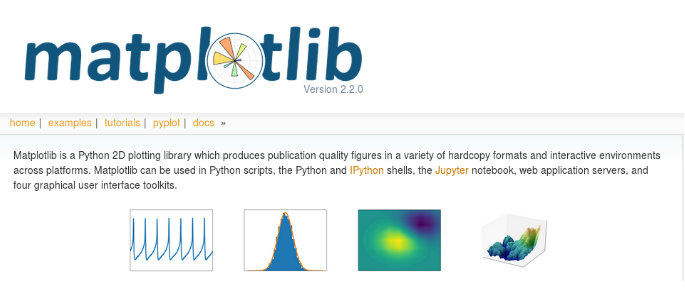To install it on an Ubuntu machine, use the following command:

`sudo apt-get install python3-matplotlib`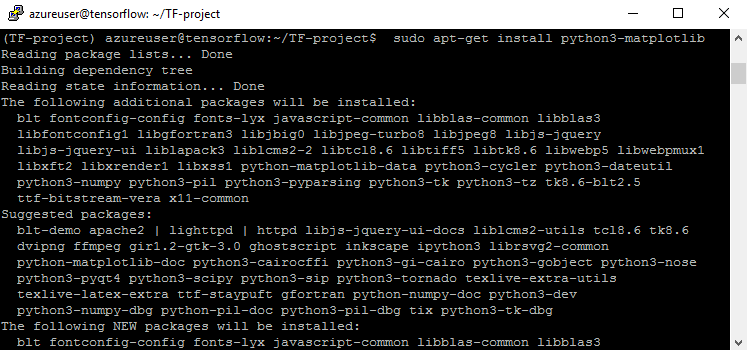To import the required packages, use import:

`import matplotlib.pyplot as pltimport numpy as np`

Use this example to prepare the data:

`x = np.linspace(0, 20, 50)`

To plot it, add this line:

`plt.plot(x, x, label='linear')`

To add a legend, use the following:

`plt.legend()`

Now, let's show the plot:

`plt.show()`

Voila! This is our plot: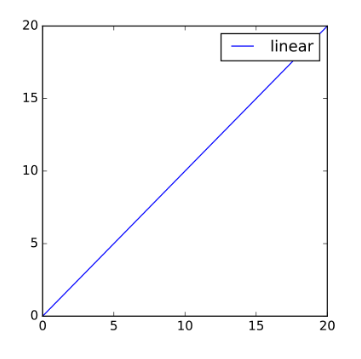# scikit-learn

I highly recommend this amazing Python library. scikit-learn is fully loaded, with various capabilities, including machine learning features. The official website of scikit-learn is http://scikit-learn.org/. To download it, use PIP, as previously discussed:

`pip install -U scikit-learn`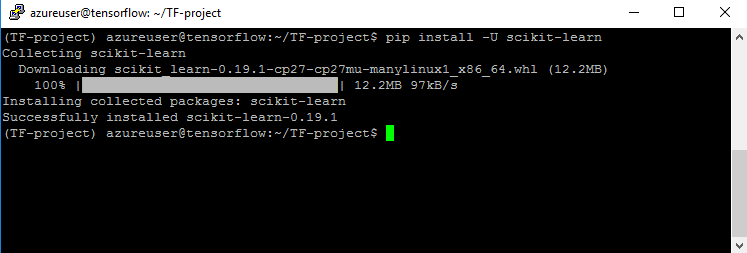# NLTK

Natural language processing is one of the most used applications in machine learning projects. NLTK is a Python package that helps developers and data scientists manage and manipulate large quantities of text. NLTK can be installed by using the following command:

`pip install -U nltk`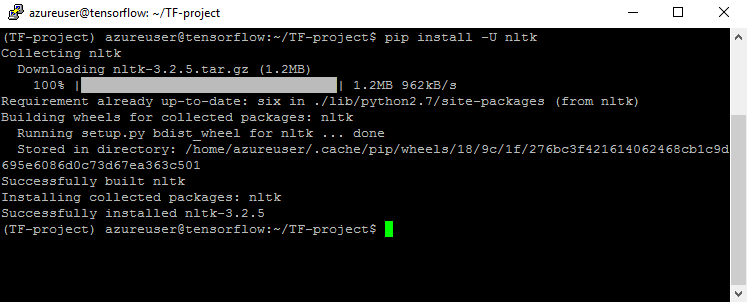Now, import nltk:

`>>> import nltk`

Install nltk packages with:

`> nltk.download()`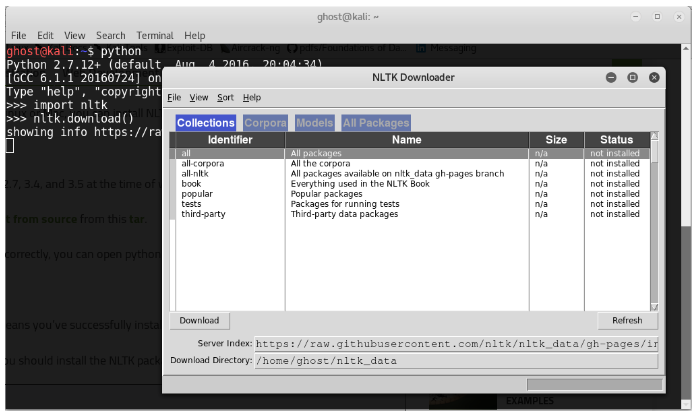You can install all of the packages: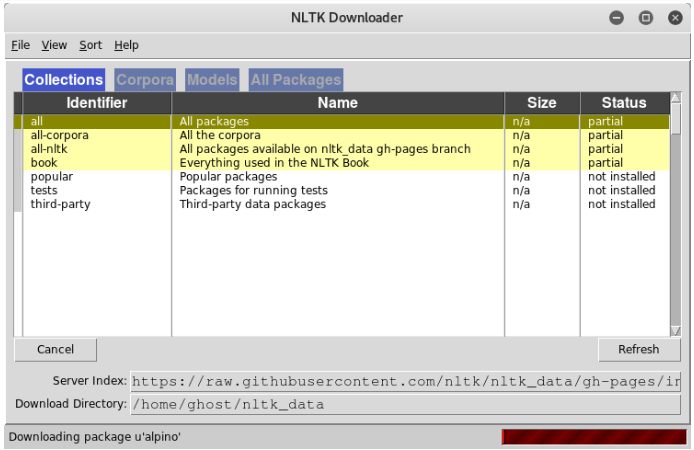If you are using a command-line environment, you just need to follow the steps: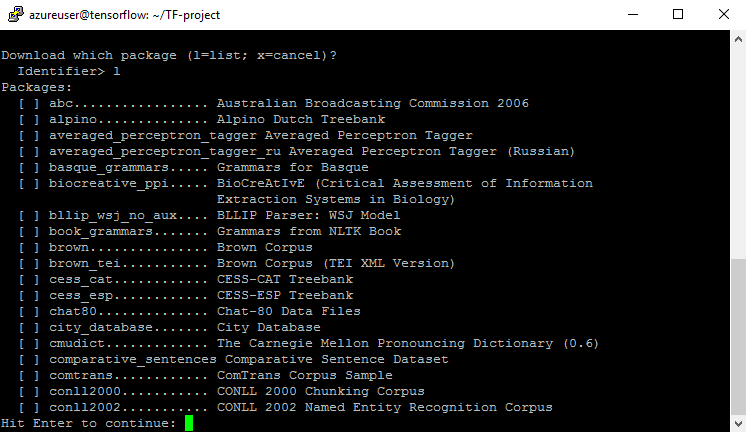If you hit all, you will download all of the packages: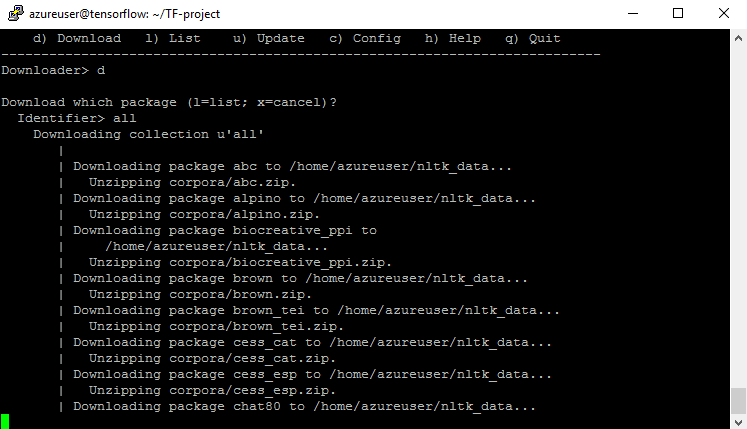# Theano

Optimization and speed are two key factors to building a machine learning model. Theano is a Python package that optimizes implementations and gives you the ability to take advantage of the GPU. To install it, use the following command:

` pip install theano`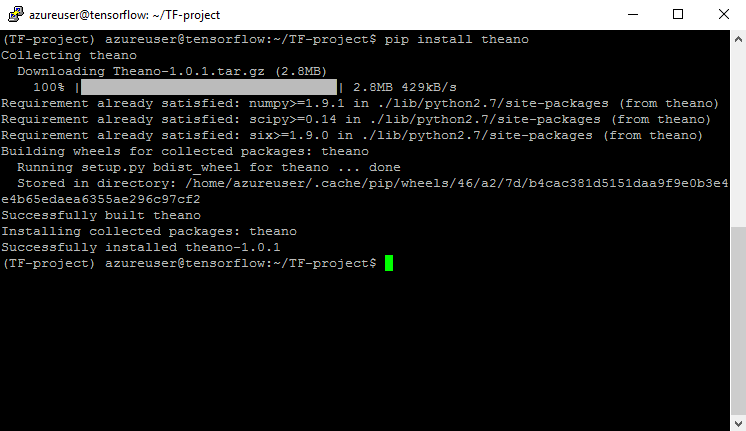To import all Theano modules, type:

`>>> from theano import *`

Here, we imported a sub-package called tensor:

`>>> import theano.tensor as T`

Let's suppose that we want to add two numbers:

`>>> from theano import function>>> a = T.dscalar('a')>>> b = T.dscalar('b')>>> c = a + b>>> f = function([a, b], c)`

The following are the full steps: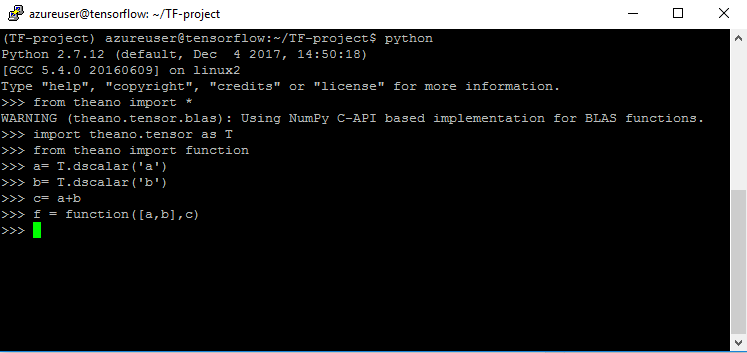By now, we have acquired the fundamental skills to install and use the most common Python libraries used in machine learning projects. I assume that you have already installed all of the previous packages on your machine. In the subsequent chapters, we are going to use most of these packages to build fully working information security machine learning projects.

# Machine learning in penetration testing - promises and challenges

Machine learning is now a necessary aspect of every modern project. Combining mathematics and cutting-edge optimization techniques and tools can provide amazing results. Applying machine learning and analytics to information security is a step forward in defending against advanced real-world attacks and threats.

Hackers are always trying to use new, sophisticated techniques to attack modern organizations. Thus, as security professionals, we need to keep ourselves updated and deploy the required safeguards to protect assets. Many researchers have shown thousands of proposals to build defensive systems based on machine learning techniques. For example, the following are some information security models:

• Supervised learning:
• Network traffic profiling
• Spam filtering
• Malware detection
• Semi-supervised learning:
• Network anomaly detection
• C2 detection
• Unsupervised learning:
• User behavior analytics
• Insider threat detection
• Malware family identification

As you can see, there are great applications to help protect the valuable assets of modern organizations. But generally, black hat hackers do not use classic techniques anymore. Nowadays, the use of machine learning techniques is shifting from defensive techniques to offensive systems. We are moving from a defensive to an offensive position. In fact, building defensive layers with artificial intelligence and machine learning alone is not enough; having an understanding of how to leverage those techniques to perform ferocious attacks is needed, and should be added to your technical skills when performing penetration testing missions. Adding offensive machine learning tools to your pentesting arsenal is very useful when it comes to simulating cutting-edge attacks. While a lot of these offensive applications are still for research purposes, we will try to build our own projects, to get a glimpse of how attackers are building offensive tools and cyber weapons to attack modern companies. Maybe you can use them later, in your penetration testing operations.

# Deep Exploit

Many great publicly available tools appeared lately that use machine learning capabilities to leverage penetration testing to another level. One of these tools is Deep Exploit. It was presented at black hat conference 2018. It is a fully automated penetration test tool linked with metasploit. This great tool uses uses reinforcement learning (self-learning).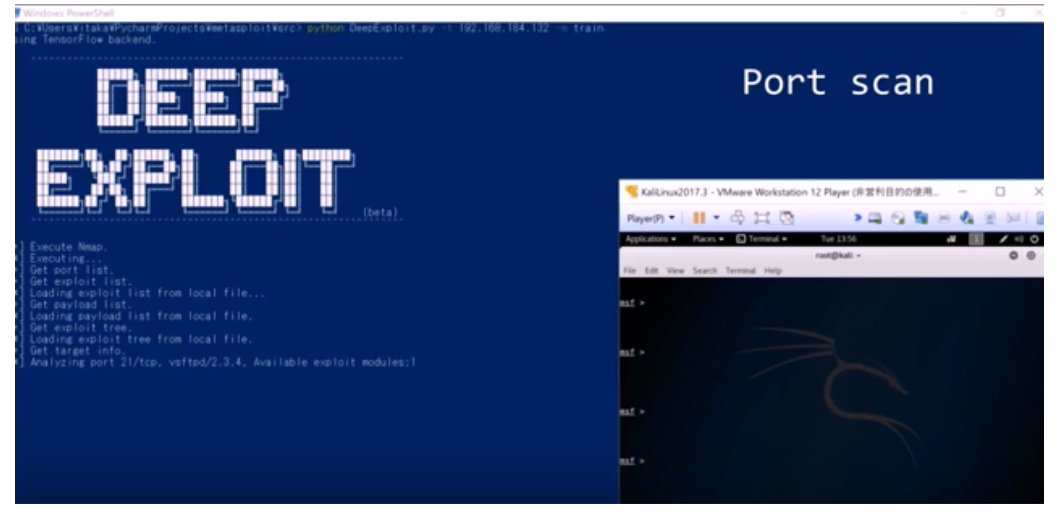It is able to perform the following tasks:

• Intelligence gathering
• Threat modeling
• Vulnerability analysis
• Exploitation
• Post-exploitation
• Reporting

To download Deep Exploit visit its official GitHub repository: https://github.com/13o-bbr-bbq/machine_learning_security/tree/master/DeepExploit.

It is consists of a machine learning model (A3C) and metasploit. This is a high level overview of Deep Exploit architecture: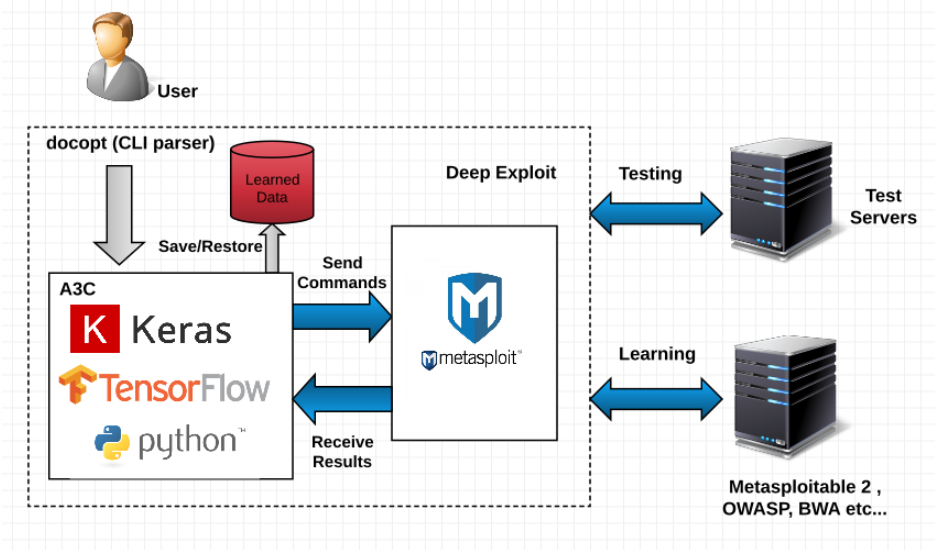The required environment to make Deep Exploit works properly is the following:

• Kali Linux 2017.3 (guest OS on VMWare)
• Memory: 8.0GB
• Metasploit framework 4.16.15-dev
• Windows 10 Home 64-bit (Host OS)
• CPU: Intel(R) Core(TM) i7-6500U 2.50GHz
• Memory: 16.0GB
• Python 3.6.1 (Anaconda3)
• TensorFlow 1.4.0
• Keras 2.1.2

# Summary

Now we have learned the most commonly used machine learning techniques; before diving into practical labs, we need to acquire a fair understanding of how these models actually work. Our practical experience will start from the next chapter.

After reading this chapter, I assume that we can build our own development environment. The second chapter will show us what it takes to defend against advanced, computer-based, social engineering attacks, and we will learn how to build a smart phishing detector. Like in every chapter, we will start by learning the techniques behind the attacks, and we will walk through the practical steps in order to build a phishing detecting system.

# Questions

1. Although machine learning is an interesting concept, there are limited business applications in which it is useful. (True | False)
2. Machine learning applications are too complex to run in the cloud. (True | False)
3. For two runs of k-means clustering, is it expected to get the same clustering results? (Yes | No)
4. Predictive models having target attributes with discrete values can be termed as:

(a) Regression models
(b) Classification models

1. Which of the following techniques perform operations similar to dropouts in a neural network?

(a) Stacking
(b) Bagging
(c) Boosting

1. Which architecture of a neural network would be best suited for solving an image recognition problem?

(a) Convolutional neural network
(b) Recurrent neural network
(c) Multi-Layer Perceptron
(d) Perceptron

1. How does deep learning differ from conventional machine learning?

(a) Deep learning algorithms can handle more data and run with less supervision from data scientists.
(b) Machine learning is simpler, and requires less oversight by data analysts than deep learning does.

(c) There are no real differences between the two; they are the same tool, with different names.

1. Which of the following is a technique frequently used in machine learning projects?

(a) Classification of data into categories.
(b) Grouping similar objects into clusters.
(c) Identifying relationships between events to predict when one will follow the other.
(d) All of the above.

To save you some effort, I have prepared a list of useful resources, to help you go deeper into exploring the techniques we have discussed.

Recommended books:

Recommended websites and online courses:

### About the Author

• ##### Chiheb Chebbi

Chiheb Chebbi is an InfoSec enthusiast who has experience in various aspects of information security, focusing on the investigation of advanced cyber attacks and researching cyber espionage and APT attacks. Chiheb is currently pursuing an engineering degree in computer science at TEK-UP university in Tunisia.

His core interests are infrastructure penetration testing, deep learning, and malware analysis. In 2016, he was included in the Alibaba Security Research Center Hall Of Fame. His talk proposals were accepted by DeepSec 2017, Blackhat Europe 2016, and many world-class information security conferences.

Browse publications by this author